Question

# Problem 2. Stored energy on a capocitor Two parallel plates are charged up by a battery...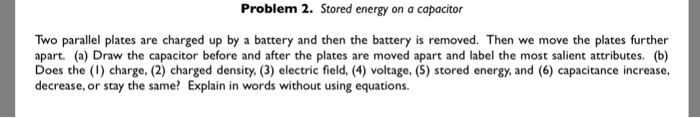Problem 2. Stored energy on a capocitor Two parallel plates are charged up by a battery and then the battery is removed. Then we move the plates further apart. (a) Draw the capacitor before and after the plates are moved apart and label the most salient attributes. (b) Does the (1) charge, (2) charged density. (3) electric field, (4) voltage, (5) stored energy, and (6) capacitance increase, decrease, or stay the same? Explain in words without using equations.

a)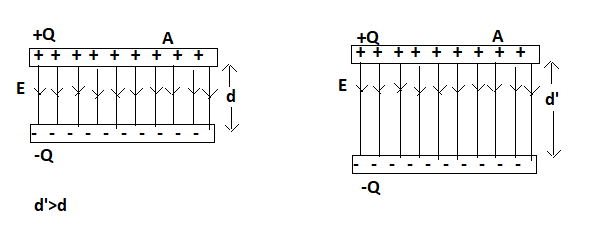b) The capacitance is charged to a charge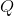then disconnected from battery.

1) Charge: As the distance between the plates is increased, chargeon the plates stays the same.

2) Charge density: Charge density is charge per unit area. Area of the plates remains the same and charge remains the same, so charge density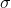also stay the same.

3) Electric field: Electric field of a parallel plate capacitor depends on charge density , so electric field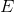also stay the same.

4)Voltage: Voltage is work done on a unit positive charge in moving it from positive plate to negative plate. As the plate seperation increases, work done also increases. So voltage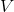between the plates also increases .

5)Stored energy: Work done in moving the capacitor plates against the electrostatic attraction is stored as energy in the capacitor. As the distance between the plates increases, potential between the plates increses and so the stored energy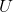also increases.

6) Capacitance: As the charge remains the same and potential between the plates increases, the capcitance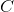of the parallel plates decreases.

#### Earn Coins

Coins can be redeemed for fabulous gifts.

Similar Homework Help Questions
• ### A parallel-plate capacitors is disconnected from a battery, and the plates are pulled a small distance...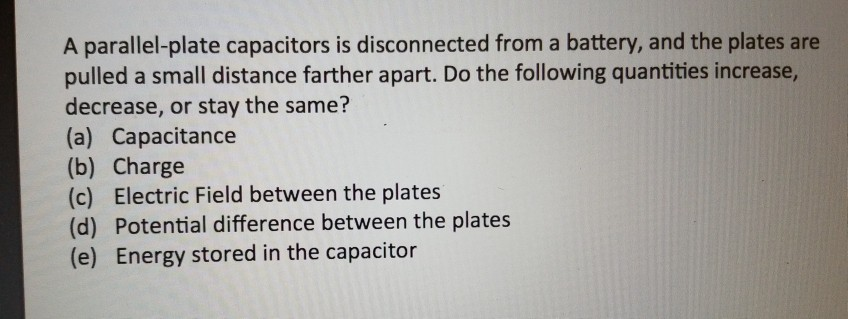A parallel-plate capacitors is disconnected from a battery, and the plates are pulled a small distance farther apart. Do the following quantities increase decrease, or stay the same? (a) Capacitance (b) Charge (c) Electric Field between the plates (d) Potential difference between the plates (e) Energy stored in the capacitor

• ### 2) A 9 volt battery is connected to a parallel plate capacitor with an initial capacitance...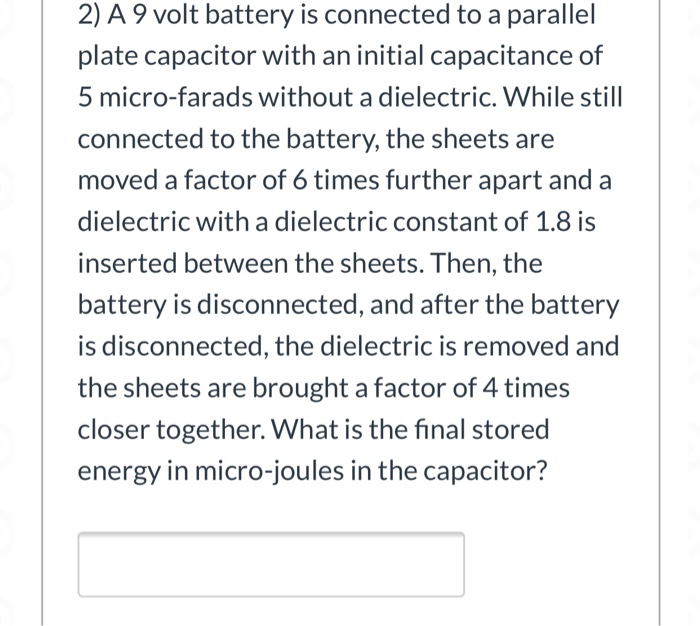2) A 9 volt battery is connected to a parallel plate capacitor with an initial capacitance of 5 micro-farads without a dielectric. While still connected to the battery, the sheets are moved a factor of 6 times further apart and a dielectric with a dielectric constant of 1.8 is inserted between the sheets. Then, the battery is disconnected, and after the battery is disconnected, the dielectric is removed and the sheets are brought a factor of 4 times closer together....

• ### A parallel plate capacitor is comprised of two metal plates with area A and separated by...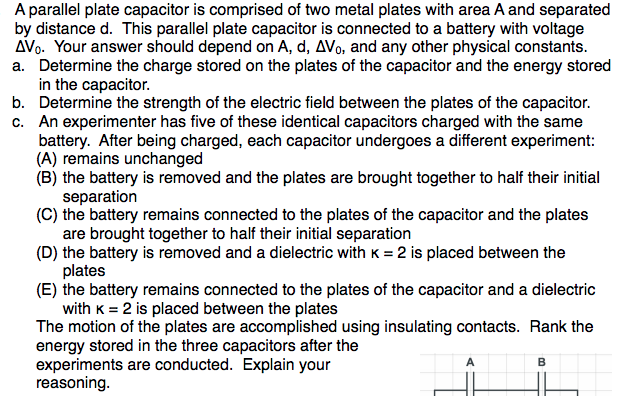A parallel plate capacitor is comprised of two metal plates with area A and separated by distance d. This parallel plate capacitor is connected to a battery with voltage AVo. Your answer should depend on A, d, ΔVo, and any other physical constants a. Determine the charge stored on the plates of the capacitor and the energy stored in the capacitor b. Determine the strength of the electric field between the plates of the capacitor c. An experimenter has five...

• ### Two rectangular parallel plates have area A and are separated by a distance d. They are...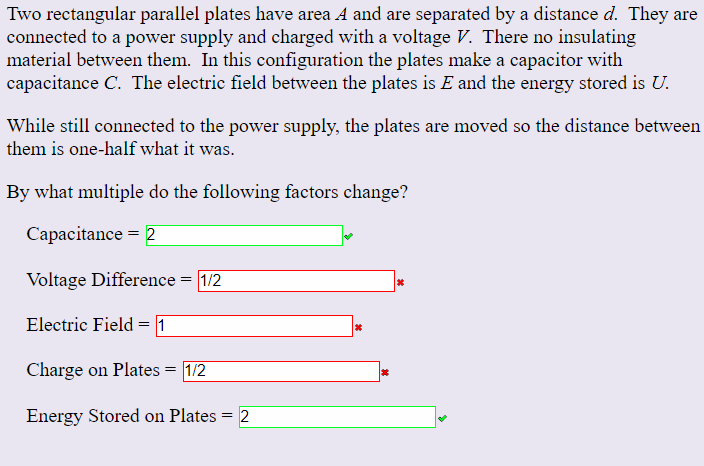Two rectangular parallel plates have area A and are separated by a distance d. They are connected to a power supply and charged with a voltage V. There no insulating material between them. In this configuration the plates make a capacitor with capacitance C. The electric field between the plates is E and the energy stored is U While still connected to the power supply, the plates are moved so the distance between them is one-half what it was. By...

• ### Two parallel plates are charged with a battery. The battery has a voltage of 12 volts...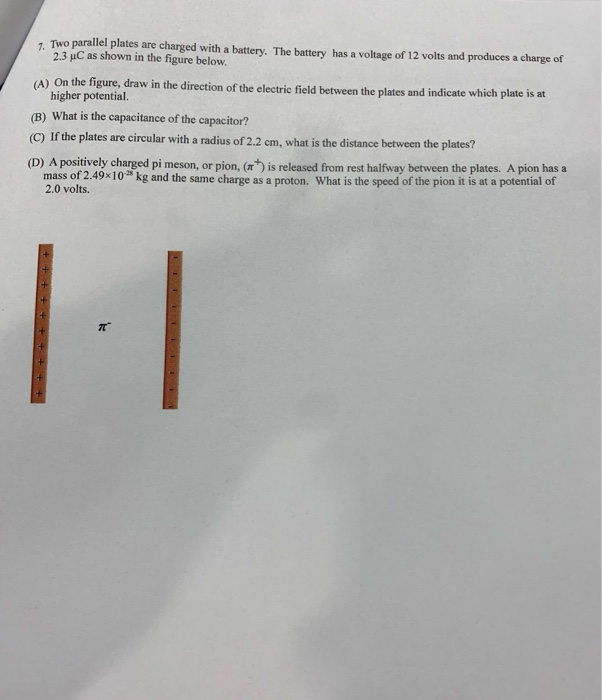Two parallel plates are charged with a battery. The battery has a voltage of 12 volts and produces a charge of 23 uC as shown in the figure below. (A) On the figure, draw in the direction of the electric field between the plates and indicate which plate is at higher potential (B) What is the capacitance of the capacitor? (C) If the plates are circular with a radius of 2.2 cm. what is the distance between the plates? (D)...

• ### 10. A parallel plate capacitor is charged up by a battery. The battery is then disconnected...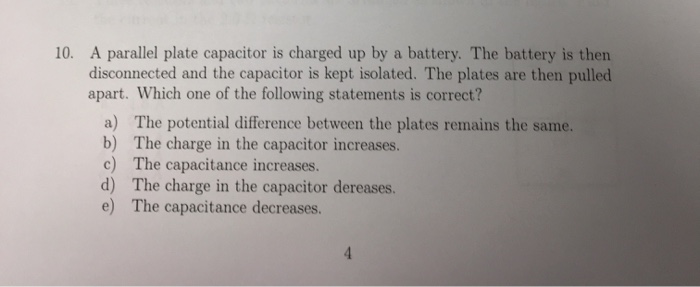10. A parallel plate capacitor is charged up by a battery. The battery is then disconnected and the capacitor is kept isolated. The plates are then pulled apart. Which one of the following statements is correct? a) The potential difference between the plates remains the same. b) The charge in the capacitor increases. c) The capacitance increases. d) The charge in the capacitor dereases. e) The capacitance decreases.

• ### A dielectric material is inserted between the charged plates of a parallel-plate capacitor. Do the following...

A dielectric material is inserted between the charged plates of a parallel-plate capacitor. Do the following quantities increase, decrease, or remain the same as equilibrium is reestablished? 1. Charge on plates (plates remain connected to battery) 2. Electric potential energy (plates isolated from battery before inserting dielectric) 3.Capacitance (plates isolated from battery before inserting dielectric) 4. Voltage between plates (plates remain connected to battery) 5. Charge on plates (plates isolated from battery before inserting dielectric) 6. Capacitance (plates remain connected...

• ### 2.(A) A capacitor consisting of two parallel plates with direct between them is connected to a battery. As a result...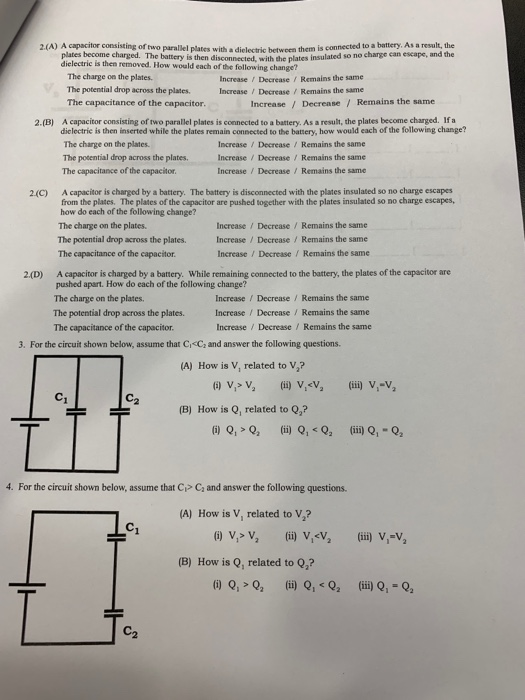2.(A) A capacitor consisting of two parallel plates with direct between them is connected to a battery. As a result, the plates become charged. The battery is then disconnected with the lates insulated so no charge can escape, and the dielectric is then removed. How would each of the following change? The charge on the plates. Increase / Decrease / Remains the same The potential drop across the plates. Increase / Decrease / Remains the same The capacitance of the...

• ### A parallel-plate vacuum capacitor is connected to a batteryand charged until the stored electric energy is...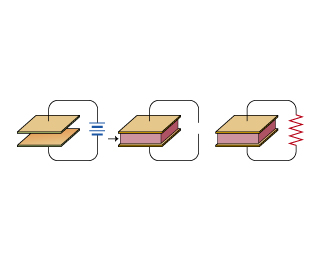A parallel-plate vacuum capacitor is connected to a battery and charged until the stored electric energy is U. The battery is removed, and then a dielectric material with dielectric constant K is inserted into the capacitor, filling the space between the plates. Finally, the capacitor is fully discharged through a resistor (which is connected across the capacitor terminals).A.)Find Ur, the the energy dissipated in the resistor.Express your answer in terms of U and other given quantities.B.) Consider the same situation...

• ### QUESTION 5 A capacitor is fully charged by a battery. When the capacitor is still connected...

QUESTION 5 A capacitor is fully charged by a battery. When the capacitor is still connected to the battery, the distance between the two plates decreases. Which of the followings will remain the same? Select all that apply. (a) voltage across the capacitor (b) capacitance (c) charge stored in the capacitor (d) electric field between the two plates (e) electric potential energy stored in the capacitor QUESTION 6 A capacitor is fully charged by a battery. When the capacitor is...